# Producing hourly temperature records for agroclimatic analysis

## The need for hourly records

Many agroclimatic models operate on an hourly basis. For tracking the transition of temperate-zone fruit trees through the dormancy season, all major chill and heat models (Anderson et al., 1986; Bennett, 1949; Erez et al., 1990; Richardson et al., 1974) require such high-resolution information. There are some models that operate on daily data (Crossa-Raynaud, 1955), but these are often just mechanisms that use proxy relationships to translate daily temperatures into what hourly-scale models would produce, if temperature data at such resolution were available. This is useful in many situations, but clearly sub-optimal, since the relationship between chill/heat and average daily temperatures (or daily extreme temperatures) varies substantially between locations. Some relationships of this nature are given in Luedeling and Brown (2011) (though these are for the ratios between chill metrics). As a consequence, computations based on hourly data are always more reliable.

Unfortunately, hourly temperature data are often not recorded in places, for which we would like to compute chill or heat accumulation. Even where records have been collected, they are often incomplete, which makes them difficult to deal with. chillR contains some functions that help in such situations. In this tutorial, we use the KA_weather and Winters_hours_gaps datasets included in chillR.

require(chillR)

All computations should also work for different datasets, as long as they are formatted as required by chillR, i.e. with columns Year, Month, Day, Tmax, Tmin for daily data, or Year, Month, Day, Hour, Temp_gaps, Temp for hourly records.

## Hourly data from daily temperature extremes

Hourly temperatures typically follow a daily temperature cycle, which can be described mathematically. This can be done in various ways that differ in accuracy and mathematical complexity. chillR implements equations provided by Linvill (1990), which are based on a sine curve for daytime temperatures, with nighttime cooling represented by a logarithmic decay function. Differences in daylength between locations are accounted for by computing sunrise and sunset times based on geographic latitude, using equations given by Spencer (1971) and Almorox et al. (2005). The results of the latter equations can be directly accessed via the daylength function, which requires only the latitude and the Julian date (day of the year) as inputs.

For example, daylength, sunrise and sunset for January 15th, for a location at latitude 50.4, can be computed by the following code:

daylength(latitude=50.4,JDay=15)
## $Sunrise ##  7.771022 ## ##$Sunset
##  16.22898
##
## $Daylength ##  8.457956 Or for the whole year: all_daylengths<-cbind(JDay=1:365,sapply(daylength(latitude=50.5,JDay=1:365),cbind)) knitr::kable(head(all_daylengths)) JDay Sunrise Sunset Daylength 1 7.962814 16.03719 8.074373 2 7.954307 16.04569 8.091387 3 7.945031 16.05497 8.109938 4 7.934996 16.06500 8.130007 5 7.924213 16.07579 8.151573 6 7.912693 16.08731 8.174613 The stack_hourly_temps applies these function, as well as those proposed by Linvill (1990) to a record of daily minimum and maximum temperatures. Before doing this, it is a good idea to apply the make_all_day_table function to ensure that no days are missing from the record (sometimes, missing data isn’t marked as NA, but represented by missing rows in a data.frame). The stack_hourly_temps requires information on the latitude: weather<-make_all_day_table(KA_weather) hourtemps<-stack_hourly_temps(weather, latitude=50.4)$hourtemps
hourtemps$DATE<-ISOdate(hourtemps$Year,hourtemps$Month,hourtemps$Day,hourtemps$Hour) This is what the resulting table looks like: DATE Year Month Day Tmax Tmin JDay Hour Temp 1998-01-01 19:00:00 1998 1 1 8.2 5.1 1 19 6.424226 1998-01-01 20:00:00 1998 1 1 8.2 5.1 1 20 6.203186 1998-01-01 21:00:00 1998 1 1 8.2 5.1 1 21 6.022921 1998-01-01 22:00:00 1998 1 1 8.2 5.1 1 22 5.870706 1998-01-01 23:00:00 1998 1 1 8.2 5.1 1 23 5.738974 1998-01-02 00:00:00 1998 1 2 9.1 5.0 2 0 5.579465 1998-01-02 01:00:00 1998 1 2 9.1 5.0 2 1 5.473672 1998-01-02 02:00:00 1998 1 2 9.1 5.0 2 2 5.378015 1998-01-02 03:00:00 1998 1 2 9.1 5.0 2 3 5.290719 1998-01-02 04:00:00 1998 1 2 9.1 5.0 2 4 5.210440 1998-01-02 05:00:00 1998 1 2 9.1 5.0 2 5 5.136133 And here is a plot of part of the data: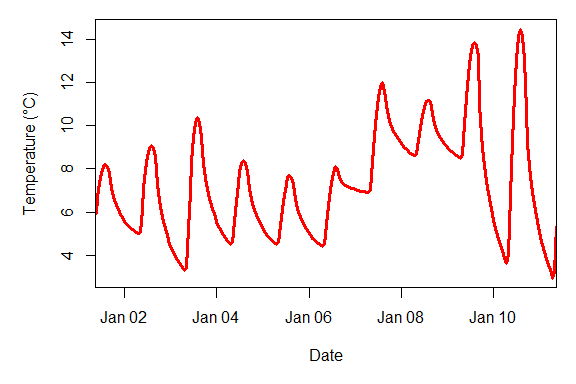This plot shows a smooth temperature curve, which can now be used for computing metrics that require such data (see below). ## Patching holes in daily temperature records Quite often, the procedure outlined above isn’t sufficent for producing a continuous record, because some daily data are missing. Let’s make such a dataset from the KA_weather data as an example: KA_weather_gaps<-KA_weather[1:100,] KA_weather_gaps[,"Tmin_original"]<-KA_weather_gaps[,"Tmin"] KA_weather_gaps[,"Tmax_original"]<-KA_weather_gaps[,"Tmax"] KA_weather_gaps$Tmin[c(4:15,20:30,35:40,44:45,48,50:60)]<-NA
KA_weather_gaps$Tmax[c(3:10,12:15,17:20,30:35,42:60,65:70)]<-NA In such cases, we have two options for fixing this situation: • we can interpolate the gaps • we can use data from another weather station to fill the gaps The first option - interpolation - is implemented in the fix_weather function: fixed<-fix_weather(KA_weather_gaps) This operation resulted in a continuous record of daily minimum and maximum temperatures, but the linearly interpolated values were not very accurate. This is shown in the figure below, where the thick blue and red lines are the actual Tmin and Tmax values and the black lines represent the interpolated values.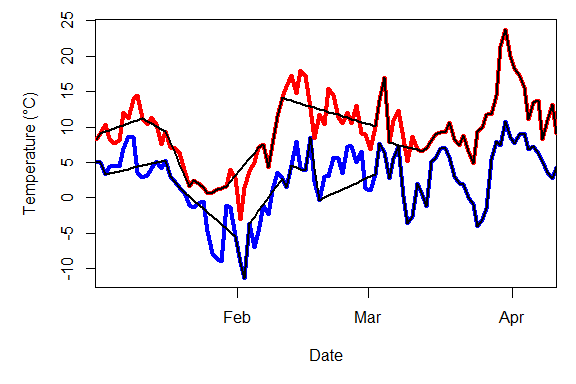For small gaps, such linear interpolation often produces accurate values, but when gaps are longer, deviation from actual values can be quite substantial. Gaps in the record can also be patched with proxy data from other weather station in the area. In this, it is critical to ensure that the proxy station is situated in a location with reasonably similar weather. Filling in gaps with records from somewhere else can easily introduce a bias - a systematic difference between stations - that could lead to large errors. These issues are addressed by the patch_daily_temperatures function, which uses one or more daily temperature datasets to fill holes in patch records. chillR contains a function that can look through the ‘Global Summary of the Day’ database and identify stations based on their coordinates. handle_gsod can do most of this work. When supplied with the action parameter ‘list_stations,’ its output is a list of weather stations in the database that are close to the specified coordinates (in c(longitude,latitude) format). To run the code below, remove the comment marks (#). Retrieving these records can sometimes take a bit of time (especially when loading many years). It also depends on internet connectivity, and on the host website still operating and using the same protocols as when the function was written. # stations<-handle_gsod(action="list_stations",location=c(6.99,50.62), # time_interval = c(1998,1998)) This list contains a column chillR_code which can be passed to the same function, when specifying the action ‘download_weather.’ Applying the same function to the resulting file then returns a dataset that chillR functions can easily use. Note that many records in this particular database have lots of gaps themselves, so it is quite common that the closest station listed there isn’t the most useful one. chillR_code STATION.NAME Lat Long distance 105180_99999 BONN-HARDTHOEHE 50.700 7.033 9.40 105170_99999 BONN/FRIESDORF(AUT) 50.700 7.150 14.39 105190_99999 BONN-ROLEBER 50.733 7.200 19.45 105130_99999 KOLN BONN 50.866 7.143 29.42 105060_99999 NUERBURG-BARWEILER 50.367 6.867 29.47 105080_99999 BLANKENHEIM 50.450 6.650 30.64 105100_99999 NUERBURG 50.333 6.950 32.05 105020_99999 NORVENICH 50.831 6.658 33.17 105140_99999 MENDIG 50.366 7.315 36.47 105090_99999 BUTZWEILERHOF(BAFB) 50.983 6.900 40.88 In this present example, which is set in Klein-Altendorf, the experimental station of the University of Bonn, Germany, only the forth-closest record (Cologne/Bonn Airport, about 30 km from the site) contained adequate data for the period of the weather record of interest. The following code accesses and processes the data for this station for the analysis. Again, uncomment the code below to actually run the functions. # patch_weather<-handle_gsod(action="download_weather",stations$chillR_code,
#                      time_interval = c(1998,1998))
# patch_weather<-handle_gsod(patch_weather)$weather Year Month Day Tmin Tmax Tmean Prec 1998 1 1 4.222222 8.722222 6.055556 4.572 1998 1 2 4.222222 9.888889 7.777778 0.254 1998 1 3 4.277778 10.888889 7.888889 3.048 1998 1 4 4.388889 9.277778 7.333333 0.000 1998 1 5 4.722222 8.777778 6.388889 0.254 Patching the gaps can then easily be done with the patch_daily_temperaturesfunction. patched_weather<-patch_daily_temperatures(KA_weather_gaps,patch_weather) The resulting list contains two elements. Let’s first look at the second one, which is called statistics: mean_bias stdev_bias filled gaps_remain Tmin 0.317 1.232 43 0 Tmax -1.001 0.928 47 0 This table contains information on the stations used for patching (only one in this case), the mean bias for Tmin and Tmax, and the bias in the standard deviation of both metrics between stations. The function automatically corrects for the mean bias, but not for the standard deviation one. The table also describes how many gaps were filled with this data, for Tmin and Tmax, respectively, and how many gaps remained. In this gaps, no gaps remained, but in other cases, it is possible to close remaining holes by adding more prpoxy stations or by using linear interpolation.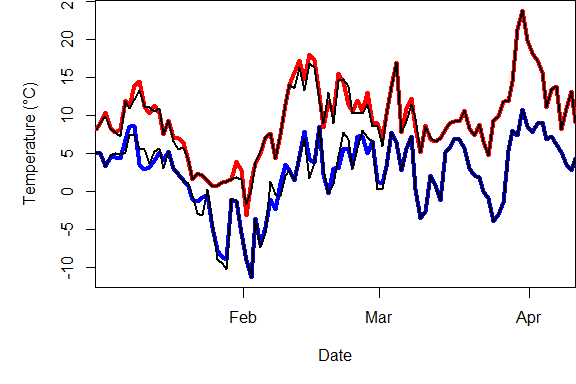Even though the original dataset of 100 days was missing 43 minimum and 47 maximum temperatures, the gaps are now barely visible, with the patched datasets corresponding quite closely to actual temperatures. These can now be translated into hourly temperatures with the stack_hourly_temps function, as described above. ## Interpolating hourly temperatures Sometimes we have actual records of hourly temperatures. While this is preferable, in principle, it may cause problems when the record isn’t complete. An example of such a record is the Winters_hours_gaps dataset contained in chillR. This is quite often the case, because temperature loggers can temporarily fail for many reasons. Gaps in such records are even harder to fill, because in this case, linear interpolation is usually not an option for gaps that span more than a couple of hours. Here’s an illustration of the error that may arise from this: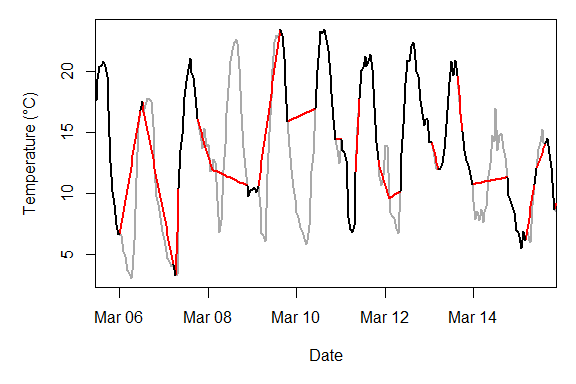In this interpolation, some daytime or nighttime cycles were missed entirely, which can lead to substantial errors when calculating agroclimatic metrics, such as chill or heat stress that are of particular concern during the warmest and coolest parts of the day. chillR’s interpolate_gaps_hourly function provides an algorithm that can produce credible and continuous hourly records from such a patchy dataset. It combines several of the elements described above, but also adds functionality to derive daily temperature extremes from hourly data that were recorded. Without going into too much detail, here is the rough mode of operation: 1. Express temperatures for each hour as a function of daily temperature extremes using the functions of Linvill (1990). According to this idealized curve, all hourly temperatures can be expressed as a function of Tmin and Tmax on the previous, same or next day of the temperature record (depending on which hour is of interest). For a day with a complete record, 24 equations can be set up. 2. For each daily temperature extreme, empirically solve the system of all equations that contain the respective Tmin or Tmax variable (this is only attempted, when a minimum of 5 equations are available, to avoid spurious results). 3. Close gaps in the resulting dataset of daily Tmin and Tmax using data from proxy stations or, as a last resort, linear interpolation. 4. Compute idealized temperature curves from the now continuous record of daily Tmin and Tmax values. 5. Calculate the difference between recorded temperatures and this idealized curve. 6. Linearly interpolate this difference and add this to the idealized temperature curve. The following code calls this function for the Winters dataset, using daily data from a nearby station of the California Irrigation Management Information System (CIMIS) as a proxy. This is retrieved with the handle_cimis function, which works similarly to the handle_gsod function described above. As before, uncomment the code to run the function (The CIMIS database seems to have occasional connectivity problems, and they’ve at least once made changes to their data storage system that required changes to the handle_cimis function. So it’s possible that the process times out or returns an error). #stations<-handle_cimis("list_stations",location=c(-122,38.5)) #downloaded_winters<-handle_cimis("download_weather",stations$chillR_code,
#               time_interval = c(2008,2008))
#winters_daily<-handle_cimis(downloaded_winters)$weather Here’s what the dataset looks like: Year Month Day Tmin Tmax Tmean Prec 2008 1 1 NA NA NA NA 2008 1 2 -1.7 13.6 5.0 0.0 2008 1 3 1.5 12.2 6.9 12.0 2008 1 4 8.0 11.0 9.2 132.8 2008 1 5 5.3 9.5 7.2 10.3 And here is the call of the interpolate_gaps_hourly function: to_interp<-Winters_hours_gaps to_interp[,"Temp_recorded"]<-to_interp[,"Temp"] to_interp[,"Temp"]<-to_interp[,"Temp_gaps"] interp<-interpolate_gaps_hourly(hourtemps=to_interp,latitude=38.5, daily_temps=list(Winters=winters_daily)) The resulting dataset has two elements: $weather and daily_patch_report. Let’s first look at the daily_patch_report element:

Var Proxy mean_bias stdev_bias filled gaps_remain
Tmin solved NA NA 193 61
Tmin Winters -0.484 2.108 61 0
Tmin interpolated NA NA 0 0
Tmax solved NA NA 202 52
Tmax Winters -1.732 2.374 52 0
Tmax interpolated NA NA 0 0

This table contains information on how many gaps in the daily record were filled by solving the system of hourly equations (‘solved’), how many Tmin and Tmax values were derived from proxy stations (listed by name, if names were provided in the call to interpolate_gaps_hourly; otherwise as station_x), and how many were filled by linear interpolation (this option can be turned off using the interpolate_remaining parameter). For proxy stations, it also provides the bias in mean Tmin and Tmax, which has been corrected, as well as the bias in the standard deviation of Tmin and Tmax (which was not corrected).

The $weather element of the interpolation result contains the table of interpolated temperatures. Year Month Day Hour Temp_gaps Temp 2008 3 4 15 21.867 21.867000 2008 3 4 16 21.604 21.604000 2008 3 4 17 20.079 20.079000 2008 3 4 18 17.344 17.344000 2008 3 4 19 13.930 13.930000 2008 3 4 20 11.929 11.929000 2008 3 4 21 NA 11.124127 2008 3 4 22 NA 10.543631 2008 3 4 23 NA 10.109254 2008 3 5 0 NA 9.827338 2008 3 5 1 NA 9.576612 2008 3 5 2 NA 9.384205 2008 3 5 3 NA 9.238165 2008 3 5 4 NA 9.129876 2008 3 5 5 NA 9.052919 2008 3 5 6 NA 9.002387 Here’s a plot of part of the data: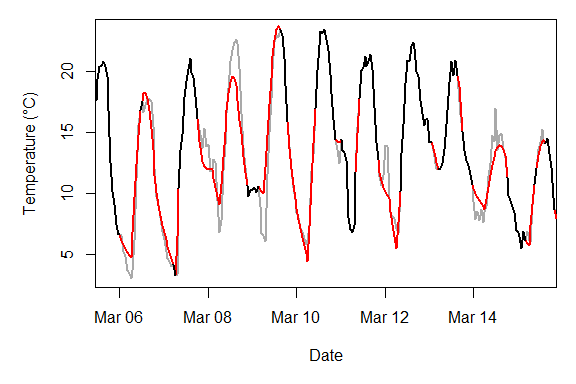This illustration shows that the interpolate_gaps_hourly function produced a pretty good approximation (red lines) to the actual temperatures (gray line). ## Accuracy assessment Since the actual hourly temperatures are known, we can evaluate the accuracy of the predictions produced by the various interpolation methods. A common measure for validating predictions is the Root Mean Square Error of the Prediction (RMSEP): $RMSEP=\sqrt{\frac{\sum_{i=1}^n(\hat{y}_i-y_i)^2}{n}}$, with $$\hat{y}_i$$ being the observed values and $$y_i$$ the predicted values. The RMSEP provides an indication of how far each predicted value deviates, on average, from the actual values. It is, however, quite difficult to interpret RMSEP values alone, because whether they indicate a good or poor model fit depends on how variable the actual values are. For instance, an RMSEP of 5 days for a phenology model (which is close to, but not quite the same as a mean error of 5 days), could indicate a very good model, if observed dates vary by several weeks or months (e.g. for bloom dates of deciduous trees), but a terrible model, if the phenological stage of interest occurs on the same day every year (e.g. the ‘phenological’ event of candles lighting up on ‘festive indoor conifers’). This is why it makes sense to include in such accuracy assessment the variation in observed values. This can be achieved by dividing the standard deviation of the observed data by the RMSEP to calculate the Residual Prediction Deviation (RPD): $RPD=\frac{sd_y}{RMSEP}$ $$\hat{y}_i$$ are the observed values, $$y_i$$ the predicted values, and $sd_y=\sqrt{\frac{\sum_{i=1}^n(y_i-\bar{y})^2}{n-1}}$ is the standard deviation, with $$\bar{y}$$ being the mean over all observations. The RPD is more useful than the RMSEP, but its use of the standard deviation can be a problem, when actual values of $$y$$ aren’t normally distributed (then the standard deviation can be a poor measure of variation). A more robust approach is use the interquartile range instead of the standard deviation. This metric is called the Ratio of Performance to InterQuartile distance (RPIQ): $RPIQ=\frac{IQ}{RMSEP}$ IQ is calculated by subtracting the 75th percentile of the distribution of all $$y$$ from the 25th percentile. require(stats) y<-rnorm(100) IQ<-quantile(y)-quantile(2) The RPIQ score is a bit harder to evaluate than the RMSEP, with different quality thresholds in use and a very high context dependency. Quite commonly, values above 2 are considered ‘good’ or even ‘excellent,’ though some studies use substantially higher thresholds (up to 8 for excellence). Since the RPIQ makes no assumption about the distribution of $$y$$, let’s use this for assessing the accuracy of the various interpolation methods. We have a total of four methods to evaluate: • idealized temperature curves from daily records of Tmin and Tmax, based on records from a nearby weather station • idealized temperature curves from daily records of Tmin and Tmax, based on records from the same location • linear interpolation of hourly temperatures • interpolation of hourly temperatures with interpolate_gaps_hourly For option 2, we first have to generate a dataset of daily minimum and maximum temperatures from the hourly records. We can do this with the make_all_day_table function (see documentation for this function for details). orchard_extremes<-make_all_day_table(inter,timestep="day", input_timestep = "hour") Let’s first look at the performance of the four methods for the periods that were missing in the hourly temperature record: winters_hours<-stack_hourly_temps(fix_weather(winters_daily),latitude=38)$hourtemps
start_hour_winters<-which(winters_hours$Year==inter$Year&
winters_hours$Month==inter$Month&
winters_hours$Day==inter$Day&
winters_hours$Hour==inter$Hour)
end_hour_winters<-which(winters_hours$Year==inter$Year[nrow(inter)]&
winters_hours$Month==inter$Month[nrow(inter)]&
winters_hours$Day==inter$Day[nrow(inter)]&
winters_hours$Hour==inter$Hour[nrow(inter)])

orchard_hours<-stack_hourly_temps(orchard_extremes,latitude=38)$hourtemps start_hour_orchard<-which(orchard_hours$Year==inter$Year& orchard_hours$Month==inter$Month& orchard_hours$Day==inter$Day& orchard_hours$Hour==inter$Hour) end_hour_orchard<-which(orchard_hours$Year==inter$Year[nrow(inter)]& orchard_hours$Month==inter$Month[nrow(inter)]& orchard_hours$Day==inter$Day[nrow(inter)]& orchard_hours$Hour==inter$Hour[nrow(inter)]) observed<-inter$Temp_recorded
option1<-winters_hours$Temp[start_hour_winters:end_hour_winters] option2<-orchard_hours$Temp[start_hour_orchard:end_hour_orchard]
option3<-interpolate_gaps(inter$Temp_gaps)$interp
option4<-inter$Temp eval_table<-eval_table_gaps<-data.frame(Option=1:4, Input_data=c("daily","daily","hourly","hourly"), Interpolation_method=c("from proxy","local extremes", "linear","hourly interpolation"), RMSEP=NA,RPIQ=NA) observed_gaps<-observed[which(is.na(inter$Temp_gaps))]
option1_gaps<-option1[which(is.na(inter$Temp_gaps))] option2_gaps<-option2[which(is.na(inter$Temp_gaps))]
option3_gaps<-option3[which(is.na(inter$Temp_gaps))] option4_gaps<-option4[which(is.na(inter$Temp_gaps))]

eval_table_gaps[,"RMSEP"]<-round(c(RMSEP(option1_gaps,observed_gaps),
RMSEP(option2_gaps,observed_gaps),
RMSEP(option3_gaps,observed_gaps),
RMSEP(option4_gaps,observed_gaps)),1)

eval_table_gaps[,"RPIQ"]<-round(c(RPIQ(option1_gaps,observed_gaps),
RPIQ(option2_gaps,observed_gaps),
RPIQ(option3_gaps,observed_gaps),
RPIQ(option4_gaps,observed_gaps)),1)

knitr::kable(eval_table_gaps,row.names = FALSE)
Option Input_data Interpolation_method RMSEP RPIQ
1 daily from proxy 2.5 4.2
2 daily local extremes 2.0 5.4
3 hourly linear 5.3 2.0
4 hourly hourly interpolation 1.9 5.6

This table shows that the interpolate_gaps_hourly function produced the best results, with an RMSEP of 1.9 and an RPIQ of 5.6. It’s interesting to note that option 3, where hourly records collected in the orchard were interpolated linearly, produced the worst fit. This highlights that, at least in this case, using an idealized temperature curve to close gaps in daily temperatures from the orchard (option 2) and even from the proxy station (option 1) produced more accurate results. Naturally, the quality of the latter approach will depend on the similarity between weather at the proxy station and in the orchard (in this case, this should be quite similar).

Restricting the comparison to only the gaps in the record is a bit unfair, because of course option 3 (linear interpolation of hourly records from the orchard) are completely accurate for hours, when temperatures were recorded. So let’s also compare the relative performance of the four methods across all hours of the record.

eval_table<-data.frame(Option=1:4,
Input_data=c("daily","daily","hourly","hourly"),
Interpolation_method=c("from proxy","local extremes",
"linear","hourly interpolation"),
RMSEP=NA,RPIQ=NA)

eval_table[,"RMSEP"]<-round(c(RMSEP(option1,observed),RMSEP(option2,observed),
RMSEP(option3,observed),RMSEP(option4,observed)),1)

eval_table[,"RPIQ"]<-round(c(RPIQ(option1,observed),RPIQ(option2,observed),
RPIQ(option3,observed),RPIQ(option4,observed)),1)

knitr::kable(eval_table,row.names = FALSE)
Option Input_data Interpolation_method RMSEP RPIQ
1 daily from proxy 2.5 4.3
2 daily local extremes 1.8 6.1
3 hourly linear 3.9 2.7
4 hourly hourly interpolation 1.4 7.5

The relative performance of the methods on the whole dataset is quite similar to the previous assessment. The quality of the proxy-based idealized temperature curves went down slightly, while all other approaches saw improvements in quality (lower RMSEP and higher RPIQ). The RPIQ values for the two interpolations that were based on local data (options 2 and 4) are very high, especially for option 4, which used the interpolate_gaps_hourly function. The RPIQ score for this option almost exceeds the ‘excellence’ threshold for the most conservative RPIQ evaluation scheme that I’ve come across (8). I find this quite remarkable, given the variable nature of daily temperature fluctuations and the fact that about half of the actually recorded values were removed before running the interpolation.

In conclusion, the interpolate_gaps_hourly function provided a very good approximation of hourly temperatures for times, when no values were recorded.

## Computing agroclimatic metrics

Finally, let’s look at the implication of the choice of interpolation method on chill and heat estimates. If we’re interested in using the Dynamic Model for winter chill or the Growing Degree Hours model for heat, we can simply calculate this using the Dynamic_Model and GDH functions in chillR. For more functionality, see the chilling and particularly the tempResponse functions.

Let’s first look at the implications of method choice on chill accumulation:

option1_chill<-Dynamic_Model(option1)
option2_chill<-Dynamic_Model(option2)
option3_chill<-Dynamic_Model(option3)
option4_chill<-Dynamic_Model(option4)
observed_chill<-Dynamic_Model(observed)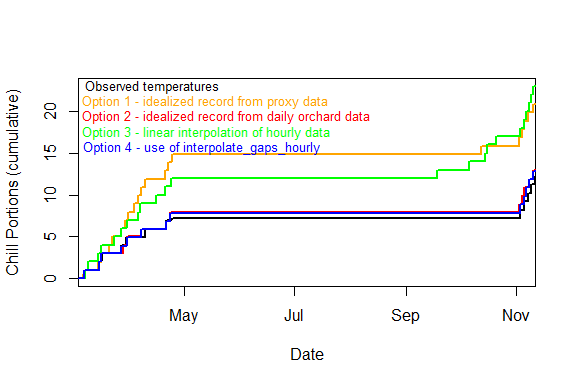This figure shows that the chill accumulation differed substantially between the options. Both the use of proxy data and the use of linear interpolation of hourly temperatures led to substantial overestimation of chill accumulation.

Here is the same assessment for heat:

option1_heat<-GDH(option1)
option2_heat<-GDH(option2)
option3_heat<-GDH(option3)
option4_heat<-GDH(option4)
observed_heat<-GDH(observed)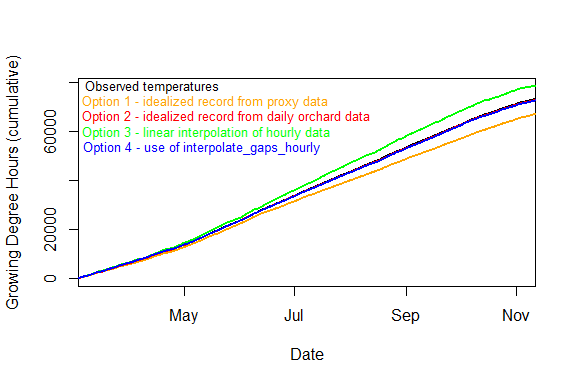This comparison doesn’t look quite as bad as for chill accumulation, but also here, option 4 clearly provided the most accurate estimate (it almost coincides with the black line, making the difference hard to see).

This dataset didn’t cover the winter season, so the chill numbers aren’t too meaningful, but it is nevertheless instructive to compare the total accumulation of chill and heat over the whole temperature record:

Option Input_data Interpolation_method Chill Portions Growing Degree Hours
0 observed none 12.2 72925
1 daily from proxy 20.9 66806
2 daily local extremes 12.9 72580
3 hourly linear 23.1 78256
4 hourly hourly interpolation 12.9 72405

This comparison shows that the choice of interpolation method can have substantial impact on our impression of accumulated chill and heat. The interpolate_gaps_hourly function in chillR outperformed all other methods evaluated here.